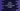# Java program to check if the first character of a string is number/digit### Java program to check if the first character of a string is number/digit:

This post will show you how to check if the first character in a string is number or digit in Java. I will show you two different ways to solve this problem : By using available methods and using Regex.

### Method 1: Using charAt and isDigit methods:

To check if the first character of a string is a number or digit, we need to :

• get the first character from the string
• check that character if it is a digit.

Java string class already has two methods that can be used to get the first character and to check if a character is digit.

To get the first character, we can use charAt method. This method is defined as below:

``public char charAt(int index)``

It returns the character if we pass the index. For the first character, we can pass 0 as the index.

To check if a character is a digit or not, we can use the below static method defined in the Character class:

``public static boolean isDigit(char ch)``

We can pass the first character and it will return one boolean value based on it is a digit or not.

#### Example program:

Let’s take a look at the below program:

``````class Main {
public static void main(String[] args) {
String[] strArray = {
"one",
"hello All",
"123 Testing !!",
"9 or 10 ?",
"\$450"
};

for (String str : strArray) {
if (Character.isDigit(str.charAt(0))) {
System.out.println("\"" + str + "\"" + " starts with a digit");
}
}
}
}``````

Here,

• strArray holds five different strings.
• Using a for loop, we are iterating through these strings.
• For each string, we are checking if the first character is digit or not by using the above two methods.
• If the return value of isDigit is true, i.e. if the first character is a digit, we are printing that string.

It prints the below output:

``````"123 Testing !!" starts with a digit
"9 or 10 ?" starts with a digit``````

### Method 2: By using regex:

We can also use a regex or regular expression. String provides one method called matches, where we can pass the regular expression and it will return one boolean value based on it matches with the string or not.

To check if the first character is digit or not, we can use ^\d.*\$ regex pattern.

It will look as like below if I change the above program to use regex :

``````class Main {
public static void main(String[] args) {
String[] strArray = {
"one",
"hello All",
"123 Testing !!",
"9 or 10 ?",
"\$450"
};

for (String str : strArray) {
if (str.matches("^\\d.*\$")) {
System.out.println("\"" + str + "\"" + " starts with a digit");
}
}
}
}``````

It will print the same output.